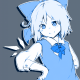• 63OR63
•Topic Author
• Offline
5 years 1 month ago - 5 years 1 month ago #1 by 63OR63
Crosshair overlay shader was created by 63OR63
Here is the rough code of my crosshair overlay for ReShade 3. It may use an external texture and apply hue/brightness/contrast/saturation adjustment to it. It may be filled with a solid color or radial gradient. It may use an inverted and oversaturated color from the background. It may have stroke. Opacity and position may be changed.
Features which I planned to implement, but haven't find a way to:
- Blurring the stroke
- Averaging the screen to use as a base color for inversion mode.
I'm abandoning the development, but maybe someone will find it interesting enough and put things in order.
```uniform float Opacity <
ui_type = "drag";
ui_min = 0.0; ui_max = 1.0;
ui_label = "Opacity";
> = 1.0;

uniform int ModPosX <
ui_type = "drag";
ui_min = -10; ui_max = 10;
ui_label = "X Axis Shift";
> = 0;

uniform int ModPosY <
ui_type = "drag";
ui_min = -10; ui_max = 10;
ui_label = "Y Axis Shift";
> = 0;

uniform bool UseStroke <
ui_type = "checkbox";
ui_label = "Stroke";
> = true;

uniform float3 StrokeColor <
ui_type = "color";
ui_label = "Stroke Color";
> = float3(0.0, 0.0, 0.0);

uniform float StrokeOpacity <
ui_type = "drag";
ui_min = 0.0; ui_max = 1.0;
ui_label = "Stroke Opacity";
> = 0.3;

uniform int XhairMode <
ui_type = "combo";
ui_label = "Xhair mode";
> = 3;

uniform float Hue <
ui_type = "drag";
ui_min = -180.0; ui_max = 180.0;
ui_label = "[Texture mode] Hue";
> = 0.0;
uniform float Brightness <
ui_type = "drag";
ui_min = -1.0; ui_max = 1.0;
ui_label = "[Texture mode] Brightness";
> = 0.0;
uniform float Contrast <
ui_type = "drag";
ui_min = 0.0; ui_max = 1.0;
ui_label = "[Texture mode] Contrast";
> = 0.0;
uniform float Saturation <
ui_type = "drag";
ui_min = 0.0; ui_max = 2.0;
ui_label = "[Texture mode] Saturation";
> = 1.0;

uniform float3 SolidColor <
ui_type = "color";
ui_label = "[Solid color mode] Color";
> = float3(0.0, 1.0, 1.0);

ui_type = "color";
ui_label = "[Gradient mode] Color 1";
> = float3(0.0, 1.0, 0.0);

ui_type = "color";
ui_label = "[Gradient mode] Color 2";
> = float3(0.0, 1.0, 1.0);

uniform float ModR <
ui_type = "drag";
ui_min = 0.01; ui_max = 1.0;
> = 0.02;

texture xhairTex	<  source = "xhair.png"; > {Width = 1920; Height = 1080; Format = RGBA8;};
sampler	xhairSampler 	{ Texture = xhairTex; };

texture xhairMaskTex	<  source = "xhair_mask.png"; > {Width = 1920; Height = 1080; Format = RGBA8;};

float3x3 QuaternionToMatrix(float4 quat)
{
float3 cross = quat.yzx * quat.zxy;
float3 square= quat.xyz * quat.xyz;
float3 wimag = quat.w * quat.xyz;

square = square.xyz + square.yzx;

float3 diag = 0.5 - square;
float3 a = (cross + wimag);
float3 b = (cross - wimag);

return float3x3(
2.0 * float3(diag.x, b.z, a.y),
2.0 * float3(a.z, diag.y, b.x),
2.0 * float3(b.y, a.x, diag.z));
}

float4 ColorShift(float4 outputColor, float H, float B, float C, float S)
{
float3 intensity;
float3 root3 = float3(0.57735, 0.57735, 0.57735);
float half_angle = 0.5 * radians(H);
float4 rot_quat = float4((root3 * sin(half_angle)), cos(half_angle));
float3x3 rot_Matrix = QuaternionToMatrix(rot_quat);
outputColor.rgb = mul(rot_Matrix, outputColor.rgb);
outputColor.rgb = (outputColor.rgb - 0.5) *(C + 1.0) + 0.5;
outputColor.rgb = outputColor.rgb + B;
float3 lumCoeff = float3(0.2125, 0.7154, 0.0721);
intensity = float(dot(outputColor.rgb, lumCoeff));
outputColor.rgb = lerp(intensity, outputColor.rgb, S);

return outputColor;
}

float4 PS_Xhair(float4 vpos : SV_Position, float2 texcoord : TEXCOORD) : SV_Target
{
float2 texcoordMod = float2(texcoord.x - ModPosX / ReShade::ScreenSize.x, texcoord.y - ModPosY / ReShade::ScreenSize.y);
float4 xhair_tex = tex2D(xhairSampler, texcoordMod);
float4 drawBackground;
float4 drawXhair;
float4 drawOpacity;

float4 outXhair;

if (XhairMode == 0)			// Texture file mode
{
xhair_tex = ColorShift(xhair_tex, Hue, Brightness, Contrast, Saturation);

drawBackground = back;
drawXhair = xhair_tex;
drawOpacity = xhair_tex.a*Opacity;
}
if (XhairMode == 1)			// Solid color mode
{
drawBackground = back;
drawXhair = float4(SolidColor, 1.0);
}
if (XhairMode == 2)			// Gradient mode
{
float4 fragColor;

float radius = sqrt(uv.x * uv.x + uv.y * uv.y) / ModR;

drawBackground = back;
drawXhair = fragColor;
}
if (XhairMode == 3)			// Inversion mode
{
float4 inv_center = 1-center;
inv_center = ColorShift(inv_center, 0, 0, 0, 180);

drawBackground = back;
drawXhair = inv_center;
}

if (UseStroke && XhairMode != 0)
{
float stroke = saturate(strokeN.a + strokeS.a + strokeW.a + strokeE.a + strokeNW.a + strokeNE.a + strokeSW.a + strokeSE.a);

float4 drawStroke = lerp(back,float4(StrokeColor,1.0),stroke*StrokeOpacity);

drawBackground = drawStroke;
}

outXhair = lerp(drawBackground,drawXhair,drawOpacity);

return outXhair;
}

technique xhair
{
pass HudPass
{
}
}```
Rename the following textures to "xhair.png" and "xhair_mask.png" accordingly:
Textures
Last edit: 5 years 1 month ago by 63OR63.
The following user(s) said Thank You: Wicked Sick, DeMondo, komaaddict, AladinZ, Gar Stazi

•• Offline
5 years 3 weeks ago #2 by AladinZ

Have a good Life.

•